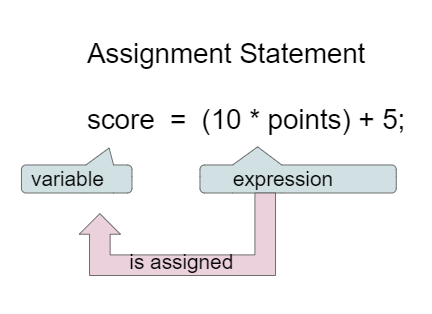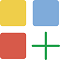# 1.4. Expressions and Assignment Statements¶

In this lesson, you will learn about assignment statements and expressions that contain math operators and variables.

## 1.4.1. Assignment Statements¶

Assignment statements initialize or change the value stored in a variable using the assignment operator =. An assignment statement always has a single variable on the left hand side. The value of the expression (which can contain math operators and other variables) on the right of the = sign is stored in the variable on the left.Figure 1: Assignment Statement (variable = expression;)¶

Instead of saying equals for the = in an assignment statement, say “gets” or “is assigned” to remember that the variable gets or is assigned the value on the right. In the figure above score is assigned the value of the expression 10 times points (which is another variable) plus 5.

The following video by Dr. Colleen Lewis shows how variables can change values in memory using assignment statements.

As we saw in the video, we can set one variable’s value to a copy of the value of another variable like `y = x;`. This won’t change the value of the variable that you are copying from.

Let’s step through the following code in the Java visualizer to see the values in memory. Click on the Next button at the bottom of the code to see how the values of the variables change. You can run the visualizer on any Active Code in this e-book by just clicking on the Code Lens button at the top of each Active Code.

Activity: CodeLens 1.4.1.2 (asgn_viz1)Check your understanding

The following has the correct code to ‘swap’ the values in x and y (so that x ends up with y’s initial value and y ends up with x’s initial value), but the code is mixed up and contains one extra block which is not needed in a correct solution. Drag the needed blocks from the left into the correct order on the right. Check your solution by clicking on the Check button. You will be told if any of the blocks are in the wrong order or if you need to remove one or more blocks. After three incorrect attempts you will be able to use the Help Me button to make the problem easier.

## 1.4.2. Adding 1 to a Variable¶

If you use a variable to keep score you would probably increment it (add one to the current value) whenever score should go up. You can do this by setting the variable to the current value of the variable plus one (score = score + 1) as shown below. The formula looks a little crazy in math class, but it makes sense in coding because the variable on the left is set to the value of the arithmetic expression on the right. So, the score variable is set to the previous value of score + 1.

Try the code below to see how score is incremented by 1. Try substituting 2 instead of 1 to see what happens.

## 1.4.3. Input with Variables¶

Variables are a powerful abstraction in programming because the same algorithm can be used with different input values saved in variables. The code below (repl JavaIOExample) will say hello to anyone who types in their name for different name values. Click on run and then type in your name. Then, try run again and type in a friend’s name. The code works for any name: behold, the power of variables!

Although you will not be tested in the AP CS A exam on using the Java System.in and Scanner classes, learning how to do input in Java is still very useful. More information on using the Scanner class can be found here https://www.w3schools.com/java/java_user_input.asp

## 1.4.4. Operators¶

Java uses the standard mathematical operators for addition (+), subtraction (-), multiplication (*), and division (/). Arithmetic expressions can be of type int or double. An arithmetic operation that uses two int values will evaluate to an int value. An arithmetic operation that uses at least one double value will evaluate to a double value. (You may have noticed that + was also used to put text together in the input program above – more on this when we talk about strings.)

Java uses the operator == to test if the value on the left is equal to the value on the right and != to test if two items are not equal. Don’t get one equal sign = confused with two equal signs ==. They mean different things in Java. One equal sign is used to assign a value to a variable. Two equal signs are used to test a variable to see if it is a certain value and that returns true or false as you’ll see below. Use == and != only with int values and not doubles because double values are an approximation and 3.3333 will not equal 3.3334 even though they are very close.Coding Exercise:

Run the code below to see all the operators in action. Do all of those operators do what you expected? What about 2 / 3? Isn’t it surprising that it prints 0? See the note below.

Note

When Java sees you doing integer division (or any operation with integers) it assumes you want an integer result so it throws away anything after the decimal point in the answer. If you need a double answer, you should make at least one of the values in the expression a double like 2.0.

With division, another thing to watch out for is dividing by 0. An attempt to divide an integer by zero will result in an ArithmeticException error message. Try it in one of the active code windows above.

Operators can be used to create compound expressions with more than one operator. You can either use a literal value which is a fixed value like 2, or variables in them. When compound expressions are evaluated, operator precedence rules are used, so that *, /, and % are done before + and -. However, anything in parentheses is done first. It doesn’t hurt to put in extra parentheses if you are unsure as to what will be done first.Coding Exercise:

In the example below, try to guess what it will print out and then run it to see if you are right. Remember to consider operator precedence. How do the parentheses change the precedence?

## 1.4.5. The Modulo Operator¶

The percent sign operator (%) is the mod (modulo) or remainder operator. The mod operator (x % y) returns the remainder after you divide x (first number) by y (second number) so 5 % 2 will return 1 since 2 goes into 5 two times with a remainder of 1. Remember long division when you had to specify how many times one number went into another evenly and the remainder? That remainder is what is returned by the modulo operator.Figure 1: Long division showing the integer result and the remainder¶

Here is a video about mod.Coding Exercise:

In the example below, try to guess what it will print out and then run it to see if you are right.

Note

The result of x % y when x is smaller than y is always x. The value y can’t go into x at all (goes in 0 times), since x is smaller than y, so the result is just x. So if you see 2 % 3 the result is 2.Check Your Understanding

## 1.4.6.Programming Challenge : Dog Years¶In this programming challenge, you will calculate your age, and your pet’s age from your birthdates, and your pet’s age in dog years. In the code below, type in the current year, the year you were born, the year your dog or cat was born (if you don’t have one, make one up!) in the variables below. Then write formulas in assignment statements to calculate how old you are, how old your dog or cat is, and how old they are in dog years which is 7 times a human year. Finally, print it all out. If you are pair programming, switch drivers (who has control of the keyboard in pair programming) after every line of code.

Calculate your age and your pet’s age from the birthdates, and then your pet’s age in dog years.

Your teacher may suggest that you use a Java IDE like repl.it for this challenge so that you can use input to get these values using the Scanner class. Here is a repl template that you can use to get started if you want to try the challenge with input.

## 1.4.7. Summary¶

• Arithmetic expressions include expressions of type int and double.

• The arithmetic operators consist of +, -, * , /, and % (modulo for the remainder in division).

• An arithmetic operation that uses two int values will evaluate to an int value. With integer division, any decimal part in the result will be thrown away.

• An arithmetic operation that uses at least one double value will evaluate to a double value.

• Operators can be used to construct compound expressions.

• During evaluation, operands are associated with operators according to operator precedence to determine how they are grouped. (*, /, % have precedence over + and -, unless parentheses are used to group those.)

• An attempt to divide an integer by zero will result in an ArithmeticException to occur.

• The assignment operator (=) allows a program to initialize or change the value stored in a variable. The value of the expression on the right is stored in the variable on the left.

• During execution, expressions are evaluated to produce a single value.

• The value of an expression has a type based on the evaluation of the expression.

## 1.4.8. AP Practice¶

The following is a 2019 AP CSA sample question.

You have attempted of activities on this page